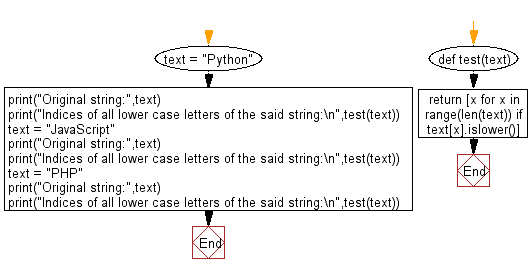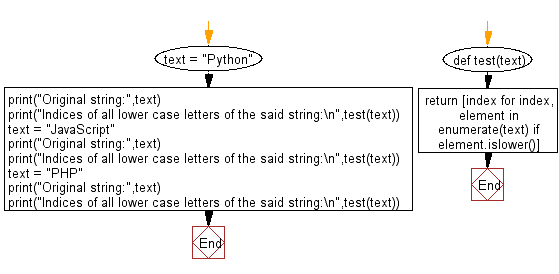﻿ Python: Index number of all lower case letters in a given string - w3resource# Python: Index number of all lower case letters in a given string

## Python Basic - 1: Exercise-129 with Solution

Write a Python program to get the index number of all lower case letters in a given string.

Sample Solution-1:

Python Code:

``````def test(text):
return [x for x in range(len(text)) if text[x].islower()]
text = "Python";
print("Original string:",text)
print("Indices of all lower case letters of the said string:\n",test(text))
text = "JavaScript";
print("Original string:",text)
print("Indices of all lower case letters of the said string:\n",test(text))
text = "PHP";
print("Original string:",text)
print("Indices of all lower case letters of the said string:\n",test(text))
``````

Sample Output:

```Original string: Python
Indices of all lower case letters of the said string:
[1, 2, 3, 4, 5]
Original string: JavaScript
Indices of all lower case letters of the said string:
[1, 2, 3, 5, 6, 7, 8, 9]
Original string: PHP
Indices of all lower case letters of the said string:
[]
```

Flowchart:Sample Solution-2:

Python Code:

``````def test(text):
return [index for index, element in enumerate(text) if element.islower()]
text = "Python";
print("Original string:",text)
print("Indices of all lower case letters of the said string:\n",test(text))
text = "JavaScript";
print("Original string:",text)
print("Indices of all lower case letters of the said string:\n",test(text))
text = "PHP";
print("Original string:",text)
print("Indices of all lower case letters of the said string:\n",test(text))
``````

Sample Output:

```Original string: Python
Indices of all lower case letters of the said string:
[1, 2, 3, 4, 5]
Original string: JavaScript
Indices of all lower case letters of the said string:
[1, 2, 3, 5, 6, 7, 8, 9]
Original string: PHP
Indices of all lower case letters of the said string:
[]
```

Flowchart:Python Code Editor:

Have another way to solve this solution? Contribute your code (and comments) through Disqus.

What is the difficulty level of this exercise?

Test your Programming skills with w3resource's quiz.

﻿

## Python: Tips of the Day

What is the difference between Python's list methods append and extend?

append: Appends object at the end.

```x = [1, 2, 3]
x.append([4, 5])
print (x)
```

Output:

```[1, 2, 3, [4, 5]]
```

extend: Extends list by appending elements from the iterable.

```x = [1, 2, 3]
x.extend([4, 5])
print (x)
```

Output:

```[1, 2, 3, 4, 5]
```

Ref: https://bit.ly/2AZ6ZFq